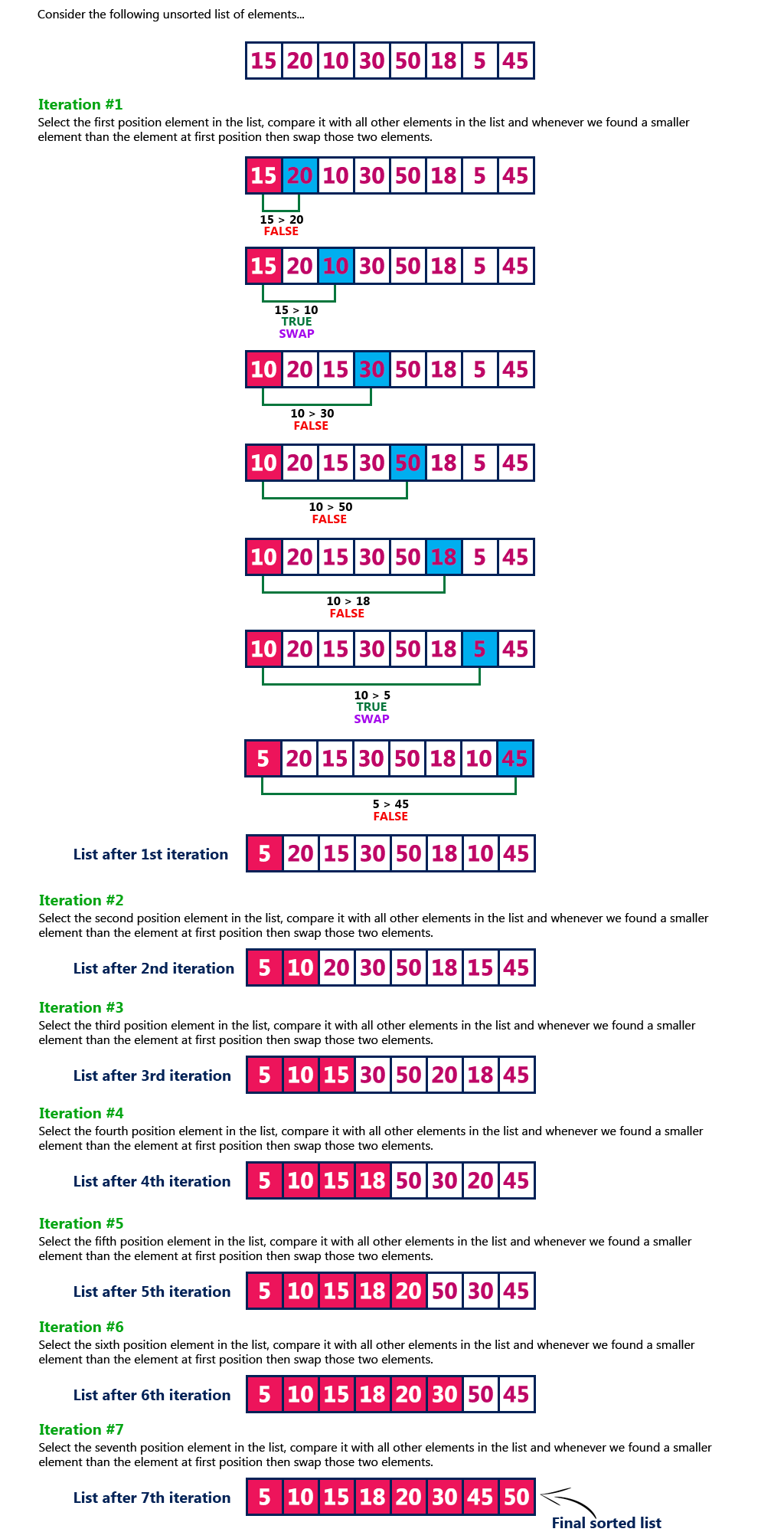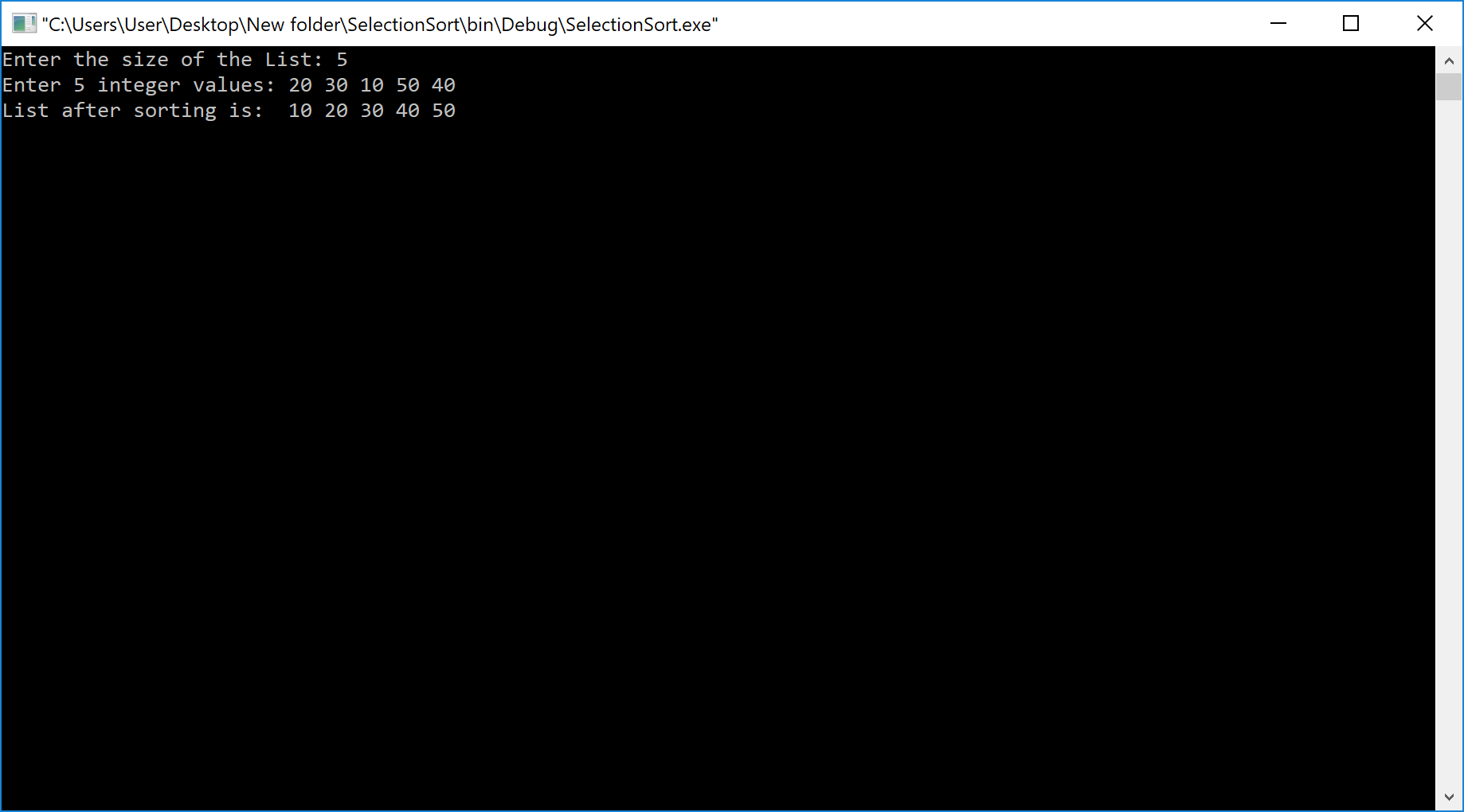The perfect place for easy learning...

×

# Selection Sort Algorithm

Selection Sort algorithm is used to arrange a list of elements in a particular order (Ascending or Descending). In selection sort, the first element in the list is selected and it is compared repeatedly with all the remaining elements in the list. If any element is smaller than the selected element (for Ascending order), then both are swapped so that first position is filled with the smallest element in the sorted order. Next, we select the element at a second position in the list and it is compared with all the remaining elements in the list. If any element is smaller than the selected element, then both are swapped. This procedure is repeated until the entire list is sorted.

Step by Step Process

The selection sort algorithm is performed using the following steps...

• Step 1 - Select the first element of the list (i.e., Element at first position in the list).
• Step 2: Compare the selected element with all the other elements in the list.
• Step 3: In every comparision, if any element is found smaller than the selected element (for Ascending order), then both are swapped.
• Step 4: Repeat the same procedure with element in the next position in the list till the entire list is sorted.

Following is the sample code for selection sort...

# Selection Sort Logic

``````//Selection sort logic

for(i=0; i<size; i++){
for(j=i+1; j<size; j++){
if(list[i] > list[j])
{
temp=list[i];
list[i]=list[j];
list[j]=temp;
}
}
}```
```

ExampleComplexity of the Selection Sort Algorithm

To sort an unsorted list with 'n' number of elements, we need to make ((n-1)+(n-2)+(n-3)+......+1) = (n (n-1))/2 number of comparisions in the worst case. If the list is already sorted then it requires 'n' number of comparisions.

Worst Case : O(n2)
Best Case : Ω(n2)
Average Case : Θ(n2)

# Implementaion of Selection Sort Algorithm using C Programming Language

``````#include<stdio.h>
#include<conio.h>

void main(){

int size,i,j,temp,list;
clrscr();

printf("Enter the size of the List: ");
scanf("%d",&size);

printf("Enter %d integer values: ",size);
for(i=0; i<size; i++)
scanf("%d",&list[i]);

//Selection sort logic

for(i=0; i<size; i++){
for(j=i+1; j<size; j++){
if(list[i] > list[j])
{
temp=list[i];
list[i]=list[j];
list[j]=temp;
}
}
}

printf("List after sorting is: ");
for(i=0; i<size; i++)
printf(" %d",list[i]);

getch();
}```
```

Output